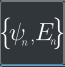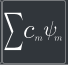# Linear Algebra

The Linear Algebra category consists of 3 nodes that relate to the spectrum of eigenstates (along with eigenvalues) and their linear combination.Spectrum

Find the spectrum of eigenstates and eigenvaluesGet Eigenvalue
Extract a single eigenvalue from the spectrumLinear Combination

Create a linear combination of eigenstates from the spectrum